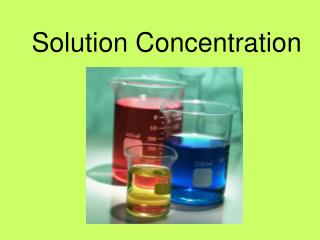Download PresentationSolution Concentration

Solution Concentration - PowerPoint PPT Presentation

Solution Concentration. Solution Concentration. Concentration : the quantity of a given solute in solution Dilute : having a relatively small quantity of solute per unit volume of solution Concentrated : having a relatively large quantity of solute per unit volume of solution.I am the owner, or an agent authorized to act on behalf of the owner, of the copyrighted work described.
Download PresentationSolution Concentration

Download Policy: Content on the Website is provided to you AS IS for your information and personal use and may not be sold / licensed / shared on other websites without getting consent from its author.While downloading, if for some reason you are not able to download a presentation, the publisher may have deleted the file from their server.

- - - - - - - - - - - - - - - - - - - - - - - - - - E N D - - - - - - - - - - - - - - - - - - - - - - - - - -
Presentation Transcript
1. Solution Concentration

2. Solution Concentration Concentration: the quantity of a given solute in solution Dilute: having a relatively small quantity of solute per unit volume of solution Concentrated: having a relatively large quantity of solute per unit volume of solution

3. In general, the concentration, “c” (lower case letter c), of any solution is expressed by the ratio quantity of solute Concentration = quantity of solvent

4. Amount Concentration • A chemists way of expressing solution concentration in terms of the amount (in moles) of solute per litre of solution • Traditionally, called molar concentration or molarity, used the symbol “M” • According to IUPAC, these terms are no longer correct and have been replaced with “amount concentration”

5. Amount Concentration • The amount concentration, c, of a solution is determined by dividing the amount (in moles) of solute, n, by the volume (in litres) of the solution, V: amountof solute (in mol) amount = concentration volume of solution (in L)

6. Weight/Volume Percent • percent (w/v) or percent (m/v) • Used with one solid and one liquid • Often used when describing unsaturated solutions mass of solute (in g) weight/volume = percent X 100% volume of solution (in mL) Example: Intravenous fluid is 0.9% (w/v) NaCl = 0.9 g of NaCl dissolved in 100 mL of solution

7. Weight/Weight Percent • weight percent, mass percent, percent (w/w) or percent (m/m) • Used with two solids or solutions of a solid dissolved in a liquid • Useful when dealing with alloys of precious metals mass of solute (in g) weight/weight = percent X 100% mass of solution (in g)

8. Weight/Weight Percent Example: The concentration of Mg2+ in seawater is 0.129% (w/w) = 0.129 g of Mg2+ dissolved in 100 g of seawater

9. Parts Per Million, Billion, and Trillion Environmental solutions are often very low concentration. Convenient to express concentrations in terms of parts per million (ppm) or parts per billion (ppb).

10. Both parts per million and parts per billion are fractions, with the mass of the solute divided by the mass of the solution ppm = mass of solute x 106 mass of solution ppb = mass of solute x 109 mass of solution

11. Calculating Concentration in ppm Health Canada’s guideline for the maximum mercury content in commercial fish is 0.5 ppm. When a 1.6 kg salmon was tested, it was found to contain 0.6 mg of mercury. Would this salmon be safe to eat?

12. ppm = mass of solute x 106 mass of solution Substitute given data. Express BOTH masses in grams allowing cancellation. ppm = 0.6 mg x 106 = 6 x 10-4 g x 106 1.6 kg 1.6x 103 g = 0.4 ppm Less than minimum guideline, therefore salmon is safe to eat.